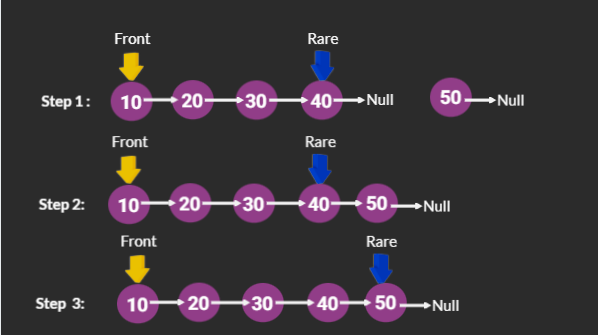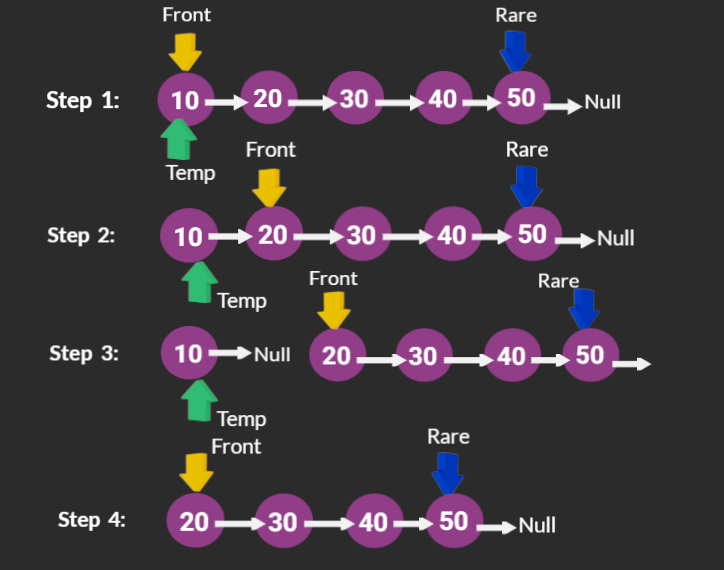# Implement Queue using Linked List

Problem Statement:  Implement Queue using Singly LinkedList

Prerequisites: Queue and LinkedList Data Structure.

Detailed Explanation of the Queue and LinkedList Data Structures is Discussed here

Queue Can be Implemented in two ways :

1. Static Implementation (Using Arrays)
2. Dynamic implementation (Using LinkedList) .

In this article, we would discuss the implementation of queue using LinkedList.

Comparison between Implementation of Queue using LinkedList and Array.

Operations Associated with queue are :

1. Enqueue     (Insert Node at Rare End )
2. Dequeue     (Delete Node from Front )
3. Peek            (Return value of Front Node without Dequing)
4. Empty         (Returns True when queue is empty else False)
5. Size             (Returns size of Queue)

Let the Initial Queue be 10→20→30→40→Null.

### Enqueue:

Let’s Enqueue Node with val 50 to Queue. Enqueue is 3 step process

• Create a node with a value that is to be Enqueued.
• Make the Rare Pointers next, point to the newly created Node.
• As the newly created Node is inserted at the rear end, this is the last value in Queue.### Dequeue :

Let’s Dequeue the front value that is, 10 from Queue.

• First create a ListNode pointer temp, and make it point to the Front value of Queue.
• We should delete the Front Value in Queue. So move the Front pointer to the next node after Front Node. That means Front = Front→next
• Temp is pointing to the previous Front value, temp→next is pointing to the newFront value, as we are interested to delete the temp, Make the temp→next point null.
• We don’t require temp anymore, So delete the temp.### Peek:

If Queue is not empty return Front value of Queue.

### Empty:

If Front is Null then Queue is empty else not.

### Size:

Maintain a variable size, initially set to zero. Upon Enqueue increment size and on Dequeue decrement size.

Code :

## C++ Code

``````#include<bits/stdc++.h>
using namespace std;

class QueueNode
{
public:
int val;
QueueNode *next;
QueueNode(int data)
{
val = data;
next = nullptr;
}
};
QueueNode *Front = nullptr, *Rare = nullptr;

class Queue
{
public:
int size = 0;
bool Empty();
void Enqueue(int value);
void Dequeue();
int Peek();
};
bool Queue ::  Empty()
{
return Front == nullptr;
}
int Queue :: Peek()
{
if(Empty())
{  cout<<"Queue is Empty"<<endl;
return -1;
}
else
return Front->val;
}
void Queue :: Enqueue(int value)
{
QueueNode *Temp;
Temp = new QueueNode(value);
if (Temp == nullptr)  //When heap exhausted
cout << "Queue is Full" << endl;
else
{
if (Front == nullptr)
{
Front = Temp;
Rare = Temp;
}
else
{
Rare->next = Temp;
Rare = Temp;
}
cout<<value <<" Inserted into Queue "<<endl;
size++;
}
}
void Queue :: Dequeue()
{
if (Front == nullptr)
cout << "Queue is Empty" << endl;
else
{
cout<<Front->val <<" Removed From Queue"<<endl;
QueueNode *Temp = Front;
Front = Front->next;
delete Temp;
size--;
}
}
int main()

{
Queue Q;
Q.Enqueue(10);
Q.Enqueue(20);
Q.Enqueue(30);
Q.Enqueue(40);
Q.Enqueue(50);
Q.Dequeue();
cout<<"The size of the Queue is "<<Q.size<<endl;
cout<<"The Peek element of the Queue is "<<Q.Peek()<<endl;
return 0;
}
``````

Output:

10 Inserted into Queue
20 Inserted into Queue
30 Inserted into Queue
40 Inserted into Queue
50 Inserted into Queue
10 Removed From Queue
The size of the Queue is 4
The Peek element of the Queue is 20

Time complexity: O(1).

Space Complexity: O(1)

## Java Code

``````import java.util.*;

class QueueNode
{
int val;
QueueNode next;
QueueNode(int data)
{
val = data;
next = null;
}
}

class Queue
{
QueueNode Front = null, Rear = null;
int size = 0;

boolean Empty()
{
return Front == null;
}
int Peek()
{
if(Empty())
{  System.out.println("Queue is Empty");
return -1;
}
else
return Front.val;
}
void Enqueue(int value)
{
QueueNode Temp;
Temp = new QueueNode(value);
if (Temp == null)  //When heap exhausted
System.out.println("Queue is Full");
else
{
if (Front == null)
{
Front = Temp;
Rear = Temp;
}
else
{
Rear.next = Temp;
Rear = Temp;
}
System.out.println(value+" Inserted into Queue ");
size++;
}
}
void Dequeue()
{
if (Front == null)
System.out.println("Queue is Empty");
else
{
System.out.println(Front.val+" Removed From Queue");
QueueNode Temp = Front;
Front = Front.next;
size--;
}
}
public static void main(String args[])

{
Queue Q=new Queue();
Q.Enqueue(10);
Q.Enqueue(20);
Q.Enqueue(30);
Q.Enqueue(40);
Q.Enqueue(50);
Q.Dequeue();
System.out.println("The size of the Queue is "+Q.size);
System.out.println("The Peek element of the Queue is "+Q.Peek());
}
}
``````

Output:

10 Inserted into Queue
20 Inserted into Queue
30 Inserted into Queue
40 Inserted into Queue
50 Inserted into Queue
10 Removed From Queue
The size of the Queue is 4
The Peek element of the Queue is 20

Time complexity: O(1).

Space Complexity: O(1)

Special thanks to SaiSri Angajala for contributing to this article on takeUforward. If you also wish to share your knowledge with the takeUforward fam, please check out this article